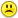+0

A bullet train left for japan at 0700. the trian stopped over at malaysia for half an hour before continuing its journey to japan . it reach

0
438
1

A bullet train left for japan at 0700. the trian stopped over at malaysia for half an hour before continuing its journey to japan . it reach japan at 1345. if the average speed of the train was 350km/h , what was the total distance covered by the train

Jul 10, 2015

#1
+8

I  think everyone drowned and the train derailed somewhere in the East China SeaI suppose it was an amphibious trainIt took 6hours and 45 minutes.  Take out the 30 min break.

That is 6 hours and 15 minutes.

That is      $$6\frac{1}{4}\;\;hours = 6.25\; hours$$

$$6.25hours\times 350km/hour$$

$${\mathtt{6.25}}{\mathtt{\,\times\,}}{\mathtt{350}} = {\frac{{\mathtt{4\,375}}}{{\mathtt{2}}}} = {\mathtt{2\,187.5}}$$

2187.5 km

Jul 10, 2015

#1
+8

I  think everyone drowned and the train derailed somewhere in the East China SeaI suppose it was an amphibious trainIt took 6hours and 45 minutes.  Take out the 30 min break.

That is 6 hours and 15 minutes.

That is      $$6\frac{1}{4}\;\;hours = 6.25\; hours$$

$$6.25hours\times 350km/hour$$

$${\mathtt{6.25}}{\mathtt{\,\times\,}}{\mathtt{350}} = {\frac{{\mathtt{4\,375}}}{{\mathtt{2}}}} = {\mathtt{2\,187.5}}$$

2187.5 km

Melody Jul 10, 2015